MathScore EduFighter is one of the best math games on the Internet today. You can start playing for free!

## Graphs to Linear Inequalities - Sample Math Practice Problems

The math problems below can be generated by MathScore.com, a math practice program for schools and individual families. References to complexity and mode refer to the overall difficulty of the problems as they appear in the main program. In the main program, all problems are automatically graded and the difficulty adapts dynamically based on performance. Answers to these sample questions appear at the bottom of the page. This page does not grade your responses.

See some of our other supported math practice problems.

### Complexity=1, Mode=simple

Write the inequality that represents this graph. Examples: y>3x/4, x<=2, y>=1, y>-2x

 1.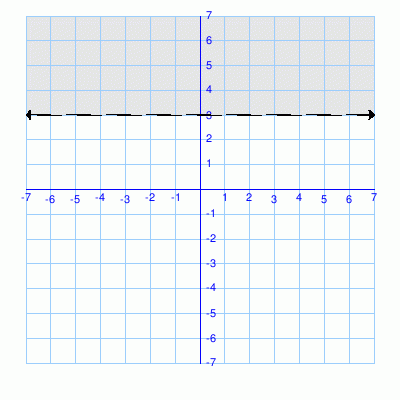Inequality: 2.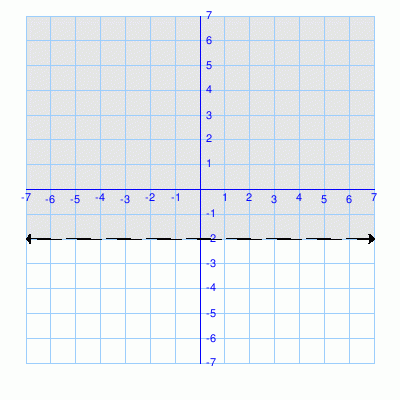Inequality:

### Complexity=1, Mode=y=mx+b

Write the inequality in y = mx + b format. Examples:   y > 2x - 3,   y ≤ -4x/3 + 1.
For less-than-or-equal-to or greater-than-or-equal-to signs, type two symbols. Example: type y <= x for y ≤ x.

 1.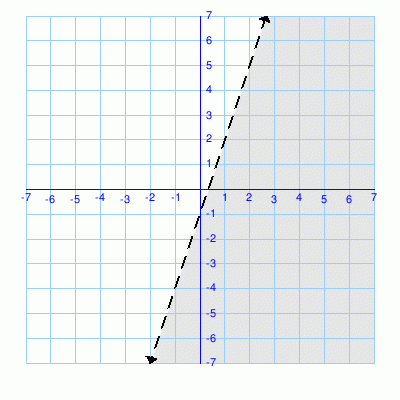Inequality: 2.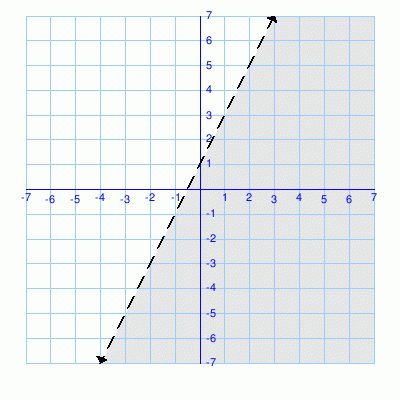Inequality:

### Complexity=2, Mode=y=mx+b

Write the inequality in y = mx + b format. Examples:   y > 2x - 3,   y ≤ -4x/3 + 1.
For less-than-or-equal-to or greater-than-or-equal-to signs, type two symbols. Example: type y <= x for y ≤ x.

 1.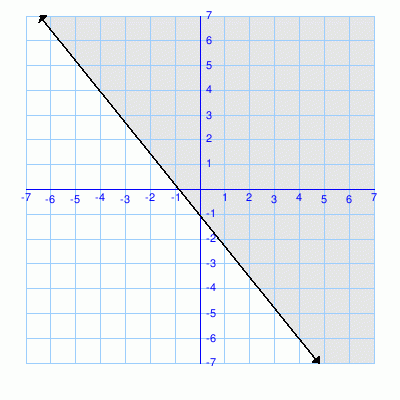Inequality: 2.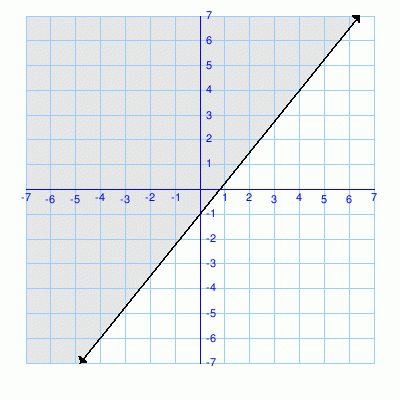Inequality:

### Complexity=1, Mode=simple

Write the inequality that represents this graph. Examples: y>3x/4, x<=2, y>=1, y>-2x

1Inequality:
Solution
Horizontal lines have a slope of 0, and are expressed in the form y=[y-intercept]
The shaded region is above the line, so we use a greater than sign.
The line is dashed, so use > for greater than.
y > 3
2Inequality:
Solution
Horizontal lines have a slope of 0, and are expressed in the form y=[y-intercept]
The shaded region is above the line, so we use a greater than sign.
The line is dashed, so use > for greater than.
y > - 2

### Complexity=1, Mode=y=mx+b

Write the inequality in y = mx + b format. Examples:   y > 2x - 3,   y ≤ -4x/3 + 1.
For less-than-or-equal-to or greater-than-or-equal-to signs, type two symbols. Example: type y <= x for y ≤ x.

1Inequality:
Solution
The slope is 3
The y intercept is -1
The shaded region is below the line, so we use a less than sign.
The line is dashed, so use < for less than.
Plugging this into y=mx+b results in y < 3x - 1
2Inequality:
Solution
The slope is 2
The y intercept is 1
The shaded region is below the line, so we use a less than sign.
The line is dashed, so use < for less than.
Plugging this into y=mx+b results in y < 2x + 1

### Complexity=2, Mode=y=mx+b

Write the inequality in y = mx + b format. Examples:   y > 2x - 3,   y ≤ -4x/3 + 1.
For less-than-or-equal-to or greater-than-or-equal-to signs, type two symbols. Example: type y <= x for y ≤ x.

1Inequality:
Solution
The slope is -5/4
The y intercept is -1
The shaded region is above the line, so we use a greater than sign.
The line is solid, so use >= for greater than or equal to.
Plugging this into y=mx+b results in

2Inequality:
Solution
The slope is 5/4
The y intercept is -1
The shaded region is above the line, so we use a greater than sign.
The line is solid, so use >= for greater than or equal to.
Plugging this into y=mx+b results in